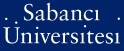# Convergence radii for eigenvalues of tri-diagonal matrices

Adduchi, Jim and Djakov, Plamen Borissov and Mityagin, Boris Samuel (2010) Convergence radii for eigenvalues of tri-diagonal matrices. Letters in Mathematical Physics, 91 (1). pp. 45-60. ISSN 0377-9017 (Print) 1573-0530 (Online)

This is the latest version of this item.Preview
PDF (This is a RoMEO green publisher -- author can archive pre-print (ie pre-refereeing) and post-print (ie final draft post-refereeing)) - Requires a PDF viewer such as GSview, Xpdf or Adobe Acrobat Reader
197Kb

Official URL: http://dx.doi.org/10.1007/s11005-009-0366-8

## Abstract

Consider a family of infinite tri-diagonal matrices of the form L + zB, where the matrix L is diagonal with entries L-kk = k(2), and the matrix B is off-diagonal, with non-zero entries B-k,B-k+1 = B-k+1,B-k = k(alpha), 0 <=alpha <= 2. The spectrum of L + zB is discrete. For small vertical bar z vertical bar the nth eigenvalue E-n(z), E-n(0)=n(2), is a well-defined analytic function. Let R-n be the convergence radius of its Taylor's series about z=0. It is proved that R-n <= C(alpha)n(2-alpha) if 0 <=alpha<11/6.

Item Type: Article tri-diagonal matrix; operator family; eigenvalues Q Science > QA Mathematics > QA299.6-433 Analysis 13811 Plamen Borissov Djakov 11 Mar 2010 10:48 25 May 2011 14:19

### Available Versions of this Item

Repository Staff Only: item control page